# What is a Fraction?

Fraction is a part of a whole.

First, I would like to make it clear that fractions are type of numbers. They are not an object, an animal, or any abstract things. They are not also shapes, tables, or any scary graphs which probably you are afraid to deal with.

They are just numbers.Now, let us go with the definition of fraction.

What does “a part of a whole” mean?

Imagine a cake. Let us slice that cake into two equal parts and let us call each part as a sliced cake.  Then, let us assume that one out of the two sliced cakes has been eaten by someone.

Questions:

1. How can we describe the amount of each sliced cake out of the whole cake?
2. How can we describe the amount of  the sliced cake which was eaten by someone?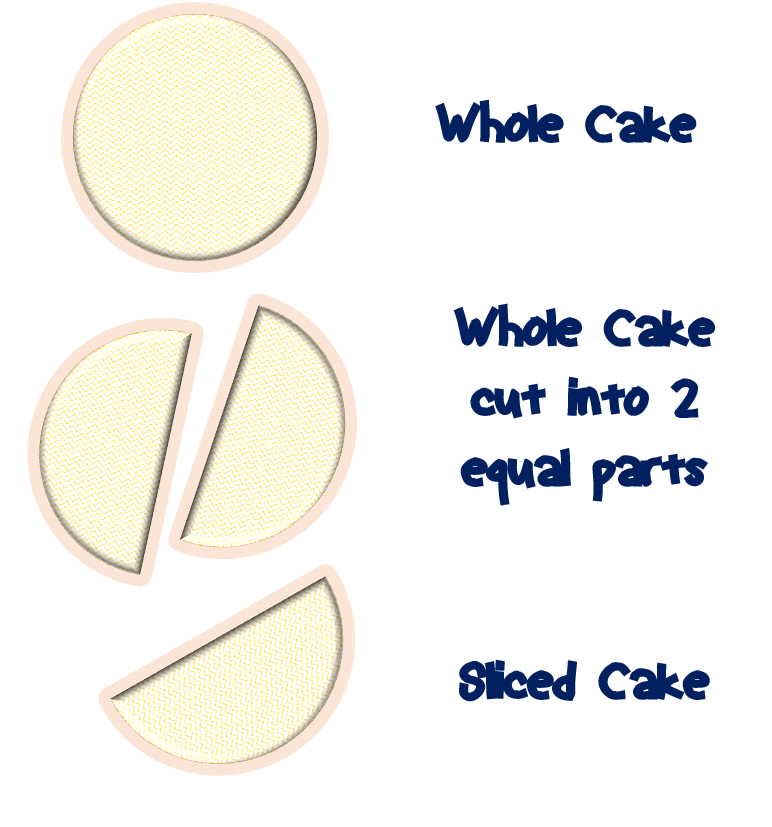Above questions can be answered by learning fractions.

By looking at the picture above, we can visualize a fraction better.

The sliced cake is actually a fraction. Then, the sliced cake which was eaten by someone is also a fraction.

The sliced cake is a fraction because it is a part of the whole cake. On the other hand, the eaten sliced cake is also a fraction because it is a part of the whole cake as well.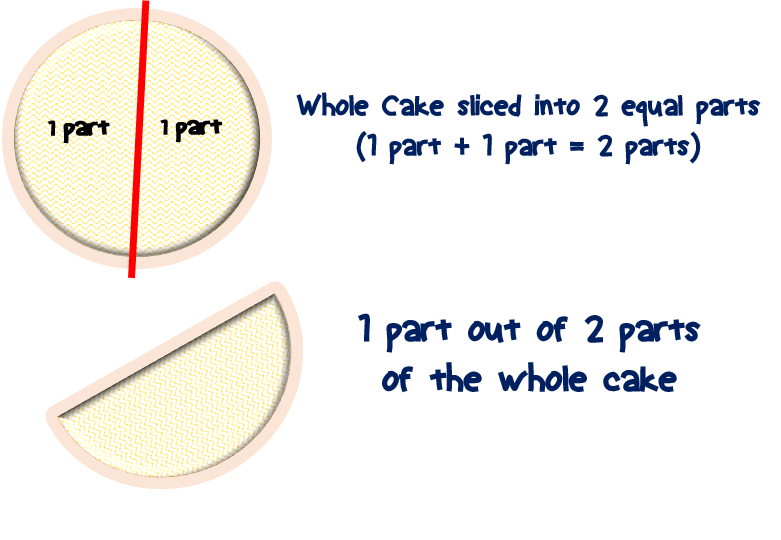Now, let us put some numbers on it.

In mathematics, the whole cake is just simply mean one unit. One unit means 1.

The sliced cakes are similarly some numbers that make up one. The eaten sliced cake is 1/2 and the remaining sliced cake is also 1/2.

We know that when we add these sliced cakes, it will make up a whole cake. It is the same as when you add 1/2 and 1/2, it will make up 1.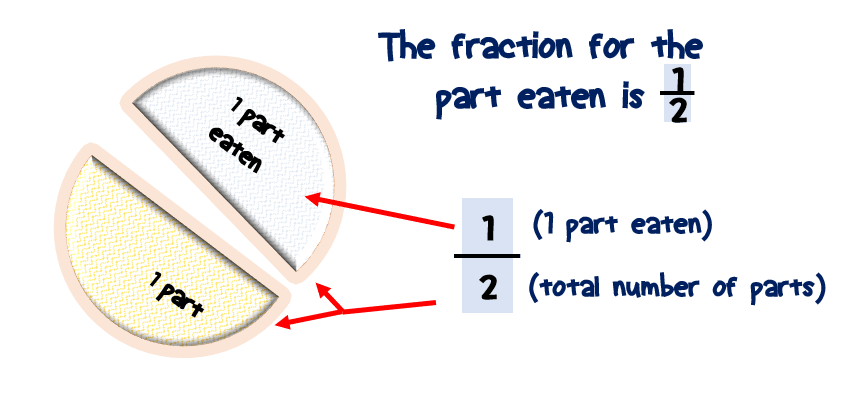Easy, isn’t it?

### How to write a fraction?

Since I already introduced to you what is a fraction, then I believe you are ready to know fraction in a more mathematical way.

Let us learn how to write a fraction,

Fraction is written with two numbers always separated by a line between those two numbers.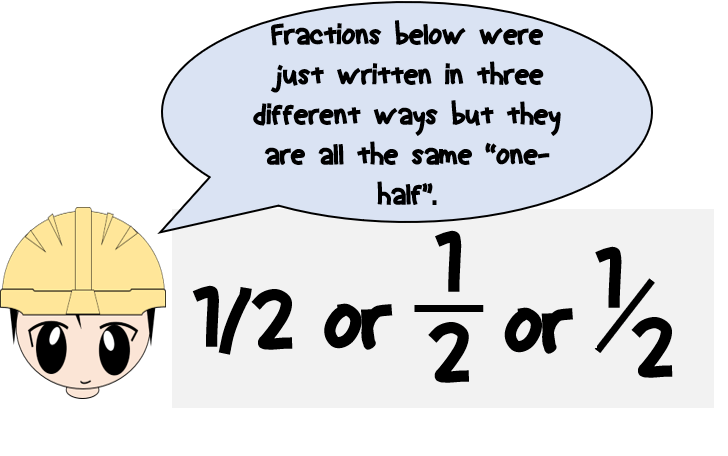For us to write a fraction better, we need to identify and name its body parts.

We can call the number above the line as the NUMERATOR.

Meanwhile, we can name the number below the line as the DENOMINATOR.

This means now that fraction has three body parts:

1. Numerator

2. Denominator

3. And one small line separating the numerator and the denominator.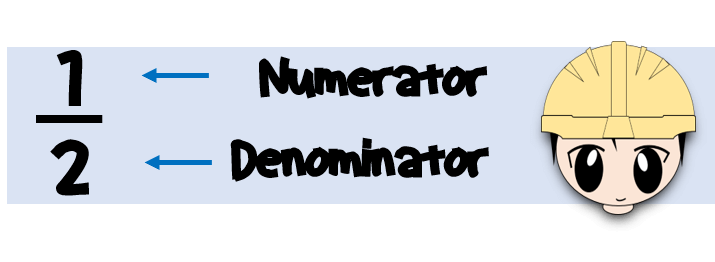Do not be intimidated by these terms. It is just their name. We will describe them later

Sometimes, people write a fraction in different ways. Do not be confused by that. As long as the three body parts of a fraction are all available, they are exactly the same. Thus, it is important not to miss in writing any of its body parts for you to call a fraction a “fraction”.

Examples.The numerator is 1 and the denominator is 2.The numerator is 1 and the denominator is 4.The numerator is 2 and the denominator is 3.The numerator is 4 and the denominator is 5.The numerator is 3 and the denominator is 10.

### How to read a fraction?

Now that we know how to write and identify fractions, Let’s learn how to read them.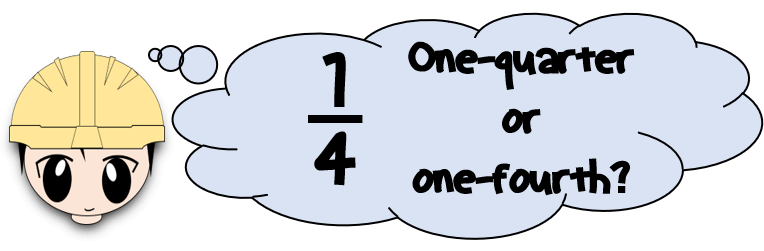Fractions can be read in different ways.

Examples.We read it as “one-half”.We read it as “one-fourth” or “one-quarter”.We read it as “two-thirds” or “two over three” or “two out of three”We read it as “four-fifth” or “four over five” or “four out of five”We read it as “three-tenth” or “three over ten”.

Let’s have further examples:We read it as “nine over one hundred”.We read it as “six over twenty-five”.

### An easy way to understand fraction.

1. How do we interpret a fraction?

Considering one unit, we can interpret fraction the same with the following examples.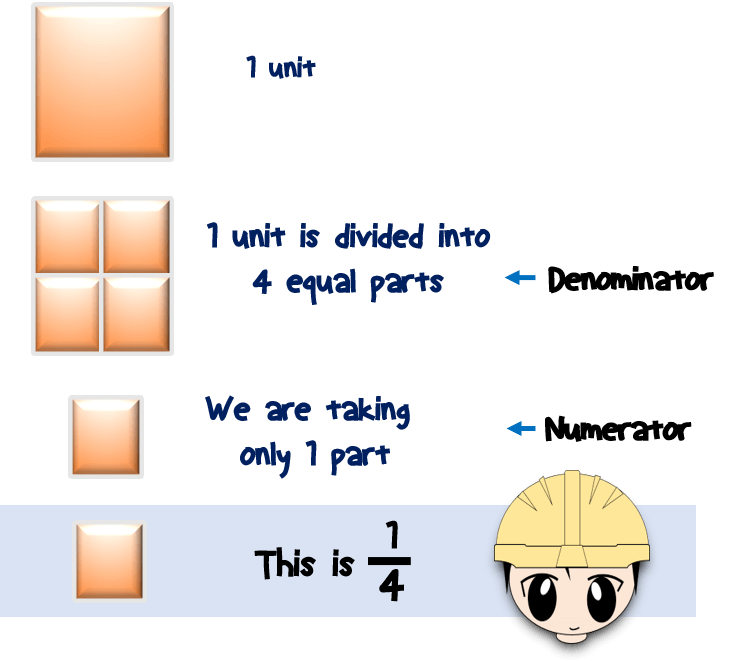It means that one unit is divided into 4 equal parts and we are taking only 1 part. In short, it means 1 part out of 4 equal parts.It means that one unit is divided into 3 equal parts and we are taking only 2 parts. In short, it means 2 parts out of 3 equal parts.It means that one unit is divided into 5 equal parts and we are taking only 4 parts. In short, it means 4 parts out of 5 equal parts.It means that one unit is divided into 10 equal parts and we are taking only 3 parts. In short, it means 3 parts out of 10 equal parts.

Thus, in an expert level of understanding, the denominator means the total number of equal parts in one unit.

And the numerator is the number of parts taken out of that total number of equal parts.

2. Another way to understand fraction.

Fraction is only a division.

It means “numerator divided by denominator”.

The numerator is the dividend and the denominator is the divisor.

The line separating the numerator and the denominator is the division operation.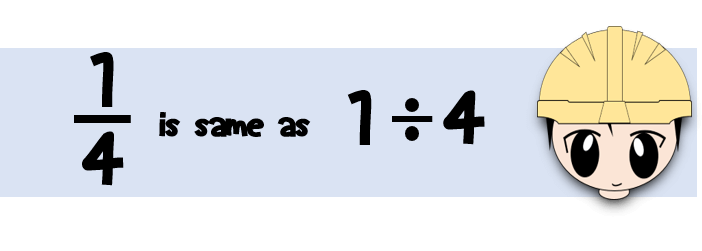Examples.

1/4 means “1 divided by 4”.

3/4 means “3 divided by 4”.

1/5 means “1 divided by 5.

2/5 means “2 divided by 5”.

2/4 means “2 divided by 4”.

### Points to Remember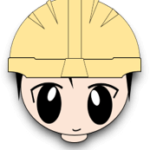Fraction is a part of a whole.

Fraction is written with two numbers separated by a line.

The number above the line is called the numerator. The number below the line is called the denominator.

The numerator is the number of parts considered or taken out of the total number. It can be interpreted also as a dividend.

The denominator is the total number of equal parts in a whole. It can be interpreted also as a divisor.

### What have you learned?

1. Explain or define what is a fraction.
2. Write and identify a fraction.
3. Read and interpret a fraction.

### Related Topics

1. What are equivalent fractions?

2. What is a proper fraction?

3. What is an improper fraction?

4. What is a mixed number?

5. How to add or subtract fractions?

6. How to simplify a fraction?

For any questions or clarifications, you can post your questions in the comments section below. You can also suggest any math related topics for my next tutorials.

1.Kelly says:

Wow! Thank you! I struggle with math so much, which means that most of the time I really don’t like it. But, this is so helpful! I’m bookmarking your page so I can refer to it again. I’ll be showing my kids this site, as well! Thank you so much!!

2.Thierry says:

Great tutorial. The post just delivered what it meant to do : understand, read and interpret a fraction. Even if I knew what a fraction is, this is a clear and efficient didactic material to teach/learn the subject. I will definitely use it with my son.
Cheers!

3.Sandra says:

Great post. I love math even though I have to look up things I don’t understand. I like that little teacher dude. Such easy understanding for kids starting fractions at school. I use fractions every time I cook.
Thank you for returning the favor.

4.Jordan says:

This clears up a lot about fractions. Seems to be a pretty huge problem area for most people, including me. So it’s good to have a clear explanation such as this.

5.Fred says:

Great way to teach fractions and easy to understand. I always loved math and did well in it through school. It takes experience and time to build up your knowledge in math but it is just fun.

1.admin says:

Thank you Fred. You can suggest or comment if something has to be changed or improve.

6.Evan Cruz says:

Great post. It really dives into what a fraction is and has clear and thorough illustrations on the subject. For future posts though, I strongly recommend that you refer to this: https://www.youtube.com/channel/UCoHhuummRZaIVX7bD4t2czg. You can thank me later!

1.admin says:

Thank you Evan.